# Higher-Degree Polynomial Functions in Trigonometry: Homework Help Chapter Exam

Exam Instructions:

Choose your answers to the questions and click 'Next' to see the next set of questions. You can skip questions if you would like and come back to them later with the yellow "Go To First Skipped Question" button. When you have completed the practice exam, a green submit button will appear. Click it to see your results. Good luck!

### Page 1

#### Question 2 2. Which of the following answer choices is a possible rational zero for the following function?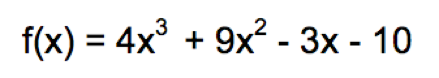#### Question 4 4. Divide using long division.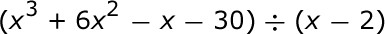### Page 2

#### Question 7 7. The following graph is of a polynomial function of degree 4. Which of the following best describes the solutions?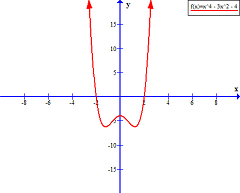#### Question 8 8. The graph of the function f(x) = x^3 - 4x^2 - 2x + 8 is shown below. This function has only one rational zero. Use the rational zeros theorem and the graph to determine the value of the rational zero.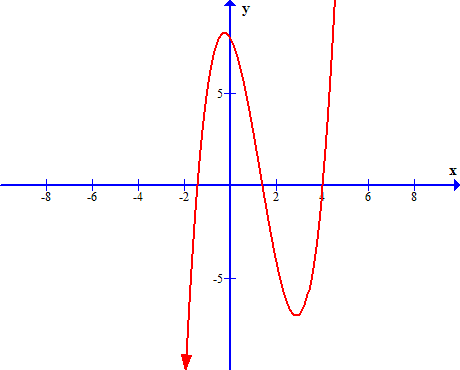#### Question 10 10. Divide the following polynomials: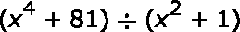### Page 3

#### Question 12 12. Divide the following polynomials: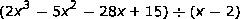### Page 4

#### Question 17 17. Divide using long division.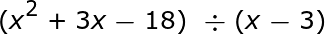#### Question 19 19. Divide using long division.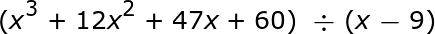### Page 5

#### Question 22 22. Divide using long division.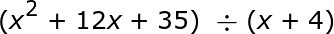#### Question 24 24. The following graph is of a polynomial function of degree 2. Are the solutions of this function real or imaginary and why?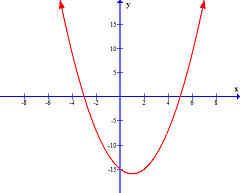### Page 6

#### Question 29 29. The possible rational zeros for the following function are +/-1 and +/-2. Which synthetic division problem shows that 2 is NOT a rational zero of the function?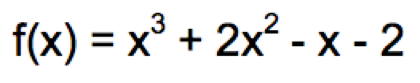#### Higher-Degree Polynomial Functions in Trigonometry: Homework Help Chapter Exam Instructions

Choose your answers to the questions and click 'Next' to see the next set of questions. You can skip questions if you would like and come back to them later with the yellow "Go To First Skipped Question" button. When you have completed the practice exam, a green submit button will appear. Click it to see your results. Good luck!

Support